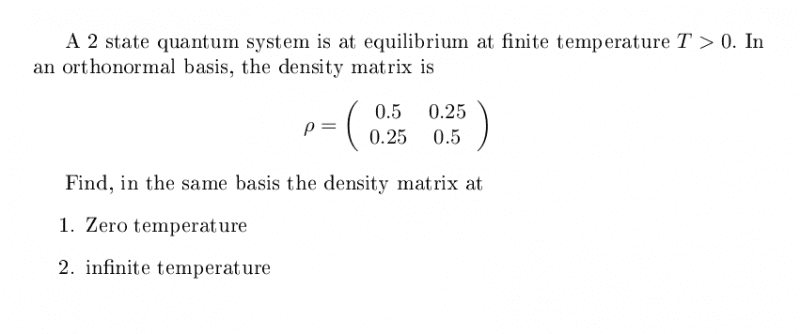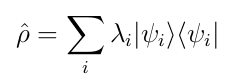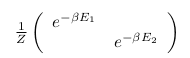# Find density matrice at zero and infinity temperature

• romeo31415

## Homework Statement## Homework Equations

Density matrix for a 2 state system is:

## The Attempt at a Solution

For a 2 finite state system, the matrice density at limit temperature is
[/B]

## The Attempt at a Solution

Last edited by a moderator:
You wrote the density matrix in terms of the energy and ##\beta##. What does the finite temperature density matrix in the problems tell you about ##E_1## and ##E_2##?

Could you give some details about how you reached the solutions you wrote?

## Homework Equations

Density matrix for a 2 state system is:## The Attempt at a Solution

For a 2 finite state system, the matrice density at limit temperature is[/B]
Evolution of the density matrix